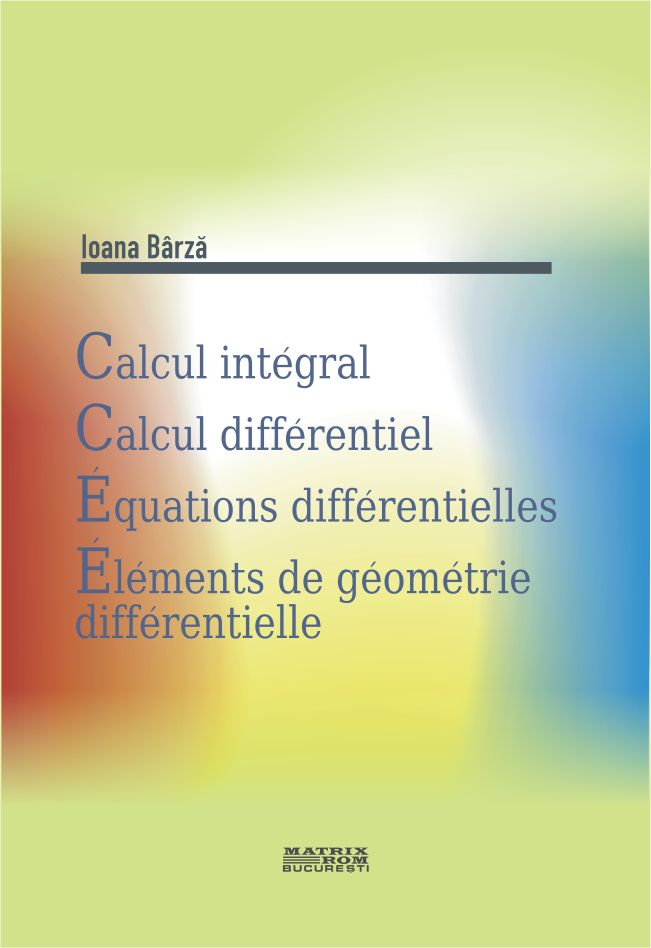Comprehensive calculation. Differential calculation. Differential equations. Elements of differential geometry | Matrix Rom
English# Comprehensive calculation. Differential calculation. Differential equations. Elements of differential geometry

Universitatea Tehnica de Constructii Bucuresti
ISBN: 978-973-755-571-7
Limba: Franceza
Suport: Hartie
97,00 lei